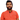# Learn Pain LessHomeOur TeamContact
Python
How to Make a Circular Color Gradient in Python: A Comprehensive GuidePawneshwer Gupta
March 05, 2023
2 min

01
Step 1: Importing the Libraries
02
Step 2: Creating the Color Gradient
03
Step 3: Displaying the Color GradientIf you’re looking to create a circular color gradient in Python, you’ve come to the right place. In this guide, we’ll take you through the steps you need to follow to create a beautiful circular color gradient using Python. This guide is about How to Make a Circular Color Gradient in Python.

At its core, a color gradient is simply a transition between two or more colors. In the case of a circular color gradient, the transition occurs in a circular fashion, with the colors blending together in a circular pattern. This effect can be used to create stunning visual effects in everything from logos to websites.

##### To create a circular color gradient in Python, we’ll need to use a few key libraries. These include:
• NumPy: NumPy is a library that provides support for large, multi-dimensional arrays and matrices. It’s an essential library for scientific computing with Python.
• Matplotlib: Matplotlib is a plotting library for Python. It provides an object-oriented API for embedding plots into applications.
• Pillow: Pillow is a fork of the Python Imaging Library (PIL). It adds support for opening, manipulating, and saving many different image file formats.

With these libraries installed, we can begin creating our circular color gradient.

## .css-1qh5hbx{box-sizing:border-box;margin:0;min-width:0;display:block;color:var(--theme-ui-colors-heading,#2d3748);font-weight:bold;-webkit-text-decoration:none;text-decoration:none;margin-bottom:1rem;font-size:1.5rem;position:relative;}Step 1: Importing the Libraries

Before we can get started, we need to import the libraries we’ll be using. Open up your Python interpreter and type in the following:

`.css-1baulvz{display:inline-block;}import numpy as npimport matplotlib.pyplot as pltfrom PIL import Image`

These three lines import the NumPy, Matplotlib, and Pillow libraries, respectively. We’ve also given them short aliases to make it easier to refer to them later on.

## Step 2: Creating the Color Gradient

Next, we need to create the actual color gradient. To do this, we’ll use NumPy to create a two-dimensional array that represents the colors we want to use in our gradient.

Here’s an example:

```width, height = 300, 300radius = min(width, height) // 2cx, cy = width // 2, height // 2
x, y = np.ogrid[:height, :width]distance_from_center = np.sqrt((x - cx) ** 2 + (y - cy) ** 2)
angle = np.arctan2(y - cy, x - cx)hue = angle / (2 * np.pi) + 0.5saturation = distance_from_center / radiusvalue = np.ones((height, width))
hsv = np.dstack((hue, saturation, value))rgb = np.squeeze(np.asarray(Image.fromarray(np.uint8(255 * hsv_to_rgb(hsv))).convert('RGB')))```

This code creates a 300x300 image with a circular color gradient. The center of the circle is located at (150, 150), and the radius of the circle is half the width of the image.

The `hsv` array contains the hue, saturation, and value for each pixel in the image. The hue is determined by the angle between the center of the circle and each pixel, while the saturation is determined by the distance from the center of the circle.

Finally, we convert the `hsv` array to an RGB array using the `hsv_to_rgb` function provided by Matplotlib. We then convert the RGB array to a PIL image and save it to a file.

## Step 3: Displaying the Color Gradient

To display the color gradient, we can use Matplotlib’s `imshow` function:

`plt.imshow(rgb)plt.show()`

This code will display the circular color gradient.

##### And that’s it! You have successfully created a circular color gradient in Python.

In conclusion, creating a circular color gradient in Python is a fun and easy way to add visual appeal to your projects. By using the Python Imaging Library, we were able to create a beautiful circular gradient with just a few lines of code.

We'll send you the best of our blog just once a month. We promise.

## Software Developer

Pawneshwer Gupta works as a software engineer who is enthusiastic in creating efficient and innovative software solutions.

Python
Flutter
Laravel
NodeJS

## Related PostsHow to Read a File in Python: A Comprehensive Guide
March 20, 2023
2 min